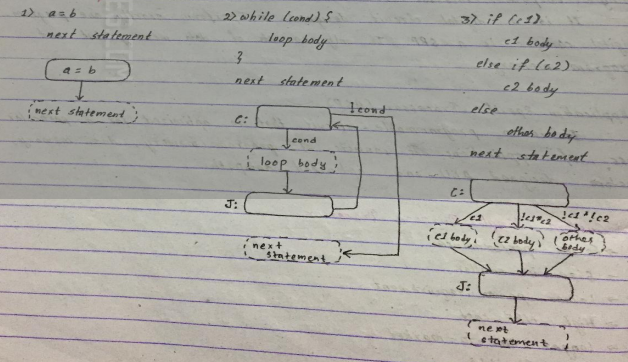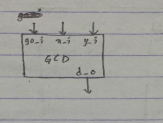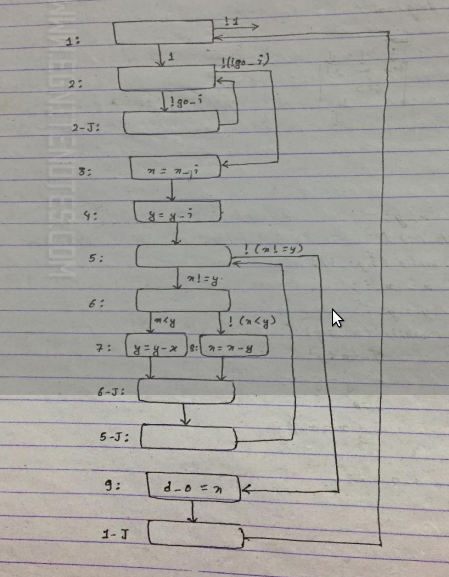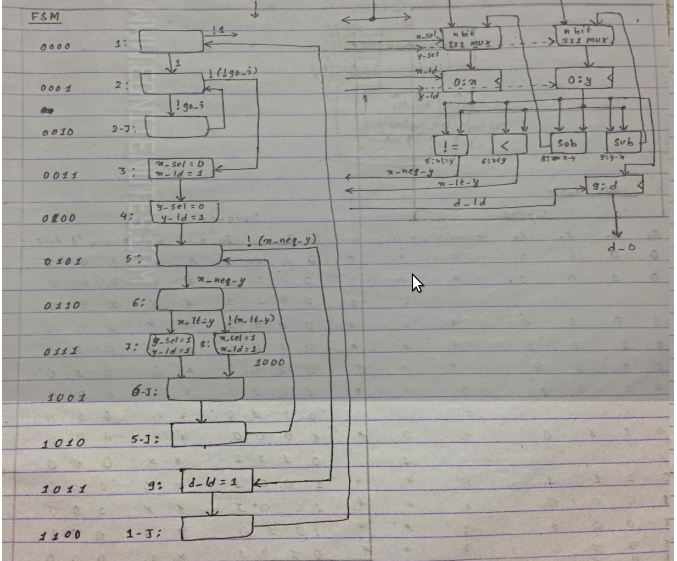### Notes of Embedded System [CT 655]

#### Hardware Design Issue

Custom Single Purpose Processor Design

Processor

Processor is a digital circuit that performs computational tasks. The minimum requirement to be a processor is the presence of controller and data path. The different processor technologies are as follows:

1. General Purpose Processor (GPP)
It is a programmable circuit that can perform varieties of tasks. It consists of program memory and general data path. The data path has large register array and one or more general purpose ALU.

2. Single Purpose Processor (SPP)
It is a digital circuit designed to perform exactly one program. Digital Camera is a SPP. It only consists of data memory but not program memory.

3. Application Specific Processor (ASP)
It is a programmable circuit that is optimized for a particular class of applications. It consists of program memory , data memory , custom designed ALU and optimized datapath.

Custom Single Purpose Processor

1. Faster performance
2. Size is smaller
3. Lower power management
4. High NRE cost
5. Longer Time to market
6. Less Flexible

Designing custom single purpose processor

1. Develop an algorithm or function that computes the desired output.
2. Convert algorithm into a complex state diagram or FSMD (finite state machine with data)
3. Divide functionality with datapath port and controller part. Datapath consists of interconnection of combinational and sequential components. Controller consists of pure FSM
4. Complete controller design by implementing FSM with combinational logic.

FSMD Templates

The templates for common algorithmic patterns are shown in figure below:Q) Greatest Common Divisor

1. Block Digaram of GCD2. Algorithm

```0: Int x,y ;
1: while(1){
2:   while(!go_i);
3:   x=x_i;
4:   y=y_i;
5:   while(x !=y){
6:     if(x7:       y=y-x;
Else
8:       x=x-y;
}
9: d_0=x ;
}```

3. State diagram or FSMD4. FSM + Data Path5. ImplementationQ) Lowest common Multiple (LCM)

LCM = (a*b)/ GCD

Algorithm:

```0: Int x,y,z
1: while(1) {
2:   while(!go_i);
3:   x=x_i;
4:   y=y_i;
5:   z=x*y;
6;   while(x!=y) {
7:     if(x8:       y=y-x;
Else
9:       x=x-y;
}
10: d_0 = z/x
}
```

Try to draw FSMD, FSM, Data path and implementation as in above example of GCD.

Q) Fibonacci series upto n places

Algorithm

```1: Int n1,n2,temp,count,n;
2: while(1) {
3:   while(!go_i);
4:   n1  = 1;
5:   n2 =1 ;
6:   n = n_i ;
7:   count = 0 ;
8:   while(count 9:     if(count !=0  && count !=1 ){
10:     temp= n1;
11:     n1 = n2 ;
12:     n2 = n1 + temp ;
}
13:   fib_0=n2;
14:   count ++ ;
}
}
```

Q) Factorial and check if prime

```Int n,f ,temp ;
while(1){
while(! go_i );
i=2;
n=n_i;
temp=n;
f=1;
while(temp!=0){
f=f*temp;
temp-- ;
}
f_0=f;
while(i		if(n%i==0)
p_0 = 0;
Else
p_0 = 1 ;
}
}```

Q)Median and variance of 5 numbers entered by user

```int  n,x1,x2,x3,x4,x5,mean,sum,i;
while(1){
while(!go_i);
n=5;
x1=x1_i;
x2=x2_i;
x3=x3_i;
x4=x4_i;
x5=x5_i;
mean = (x1+x2+x3+x4+x5)/5;
median_0=x[(n+1)/2];
sum=0;
i=0;
while(i<5){
sum=sum+ (x[i] –mean)*  (x[i] – mean);
i++;
}
Variance_0= sum/n;
}```

Optimizing Custom Single Purpose Processor

Optimization

Optimization is the technique of improving the design metrics so as to get the best possible values of various design metrics.The optimization opportunities in custom SPP are as follows:-

1. Optimization of original program:

The algorithms are analyzed in terms of time complexity and space complexity , and hence we try to develop more efficient alternative algorithms. It involves decreasing of number of computations and size of variables if possible.

E.g. Optimized GCD Program

```	int x,y,r
while(1){
while(!go_i);
if(x_i >=y_i) {
x=x_i ;
y=y_i ;
}
else {
x=y_i;
y=x_i ;
}
while(y!=0) {
x=x%y;
x=y;
y=x;
}
d_0 = x ;
}
```

2. Optimizing FSMD

The states that can be merged to reduce the number of states . The design must be aware if whether o/p timing may or may not be modified.

E.g. Optimized GCD FSMD
Draw FSMD from the above optimized algorithm of GCD.

3. Optimizing Datapath

Many functional operations can share a single functional unit if those operations occur in different stages. E.g. In GCD Datapath,single subtractor can be used and selection can be done using multiplexor.

4. Optimizing FSM

FSM can be optimized using state encoding and state minimization state encoding is the task of assigning a unique log2(n) bits to encode n states.

State minimization is the task of merging equivalent states into a single state.